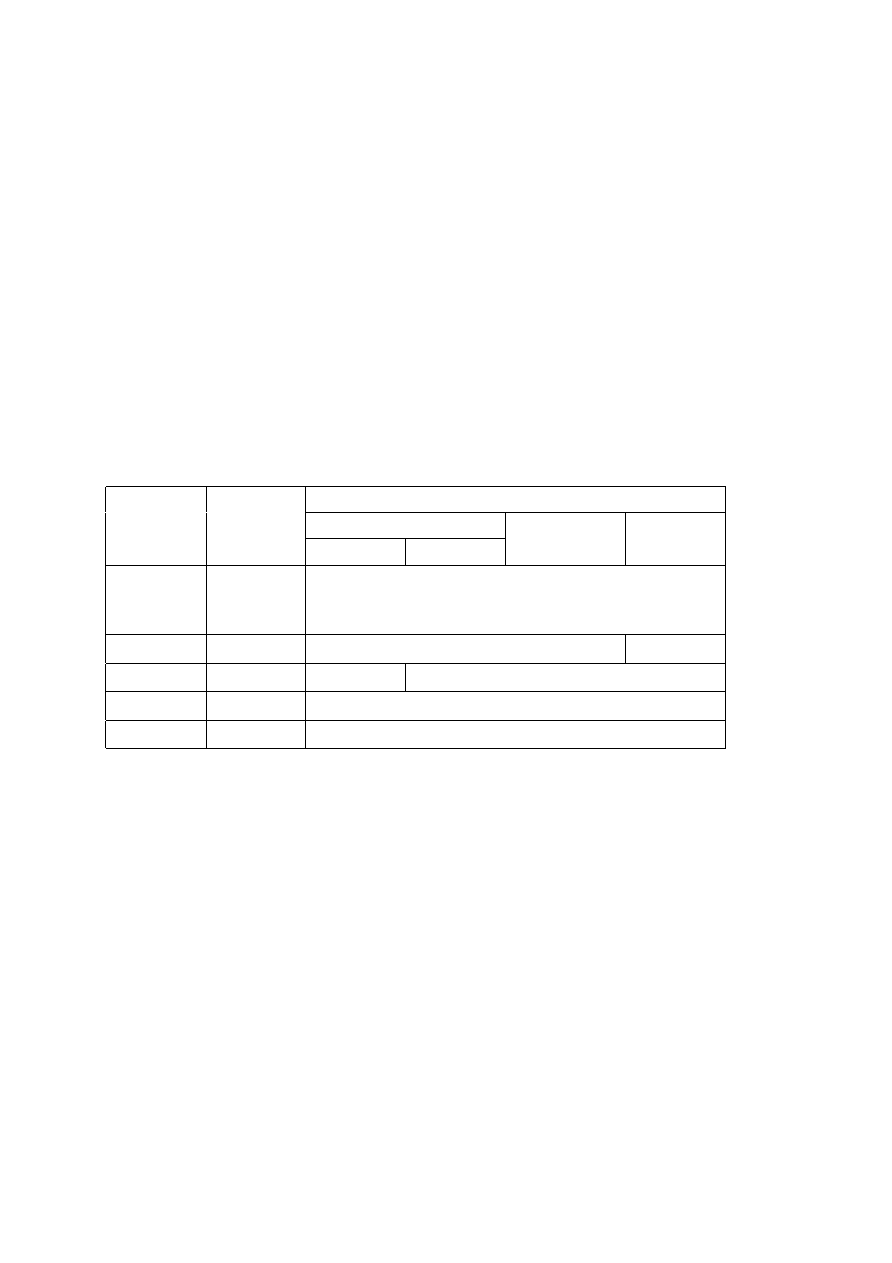ISO/IEC 10918-1 : 1993(E)
The marker and parameters shown in Figure B.7 are defined below. The size and allowed values of each parameter are
given in Table B.5.
DHT:
Define Huffman table marker ­ Marks the beginning of Huffman table definition parameters.
Lh:
Huffman table definition length ­ Specifies the length of all Huffman table parameters shown in Figure B.7
(see B.1.1.4).
Tc:
Table class ­ 0 = DC table or lossless table, 1 = AC table.
Th:
Huffman table destination identifier ­ Specifies one of four possible destinations at the decoder into which
the Huffman table shall be installed.
L
i
:
Number of Huffman codes of length i ­ Specifies the number of Huffman codes for each of the 16 possible
lengths allowed by this Specification. L
i
's are the elements of the list BITS.
V
i,j
:
Value associated with each Huffman code ­ Specifies, for each i, the value associated with each Huffman
code of length i. The meaning of each value is determined by the Huffman coding model. The V
i,j
's are the
elements of the list HUFFVAL.
Table B.5 ­ Huffman table specification parameter sizes and values
Values
Parameter
Size (bits)
Sequential DCT
Progressive DCT
Lossless
Baseline
Extended
Lh
16
2
17
1
+
+
=
t
n
t
m
?
D
Tc
1
4
0, 1
0
Th
1
4
0, 1
0-3
L
i
1
8
0-255
V
i, j
1
8
0-255
The value n in Table B.5 is the number of Huffman tables specified in the DHT marker segment. The value m
t
is the
number of parameters which follow the 16 L
i
(t) parameters for Huffman table t, and is given by:
m
L
t
i
i
=
=
1
16
In general, m
t
is different for each table.
Once a Huffman table has been defined for a particular destination, it replaces the previous tables stored in that
destination and shall be used when referenced, in the remaining scans of the current image and in subsequent images
represented in the abbreviated format for compressed image data. If a table has never been defined for a particular
destination, then when this destination is specified in a scan header, the results are unpredictable.
CCITT Rec. T.81 (1992 E)
41